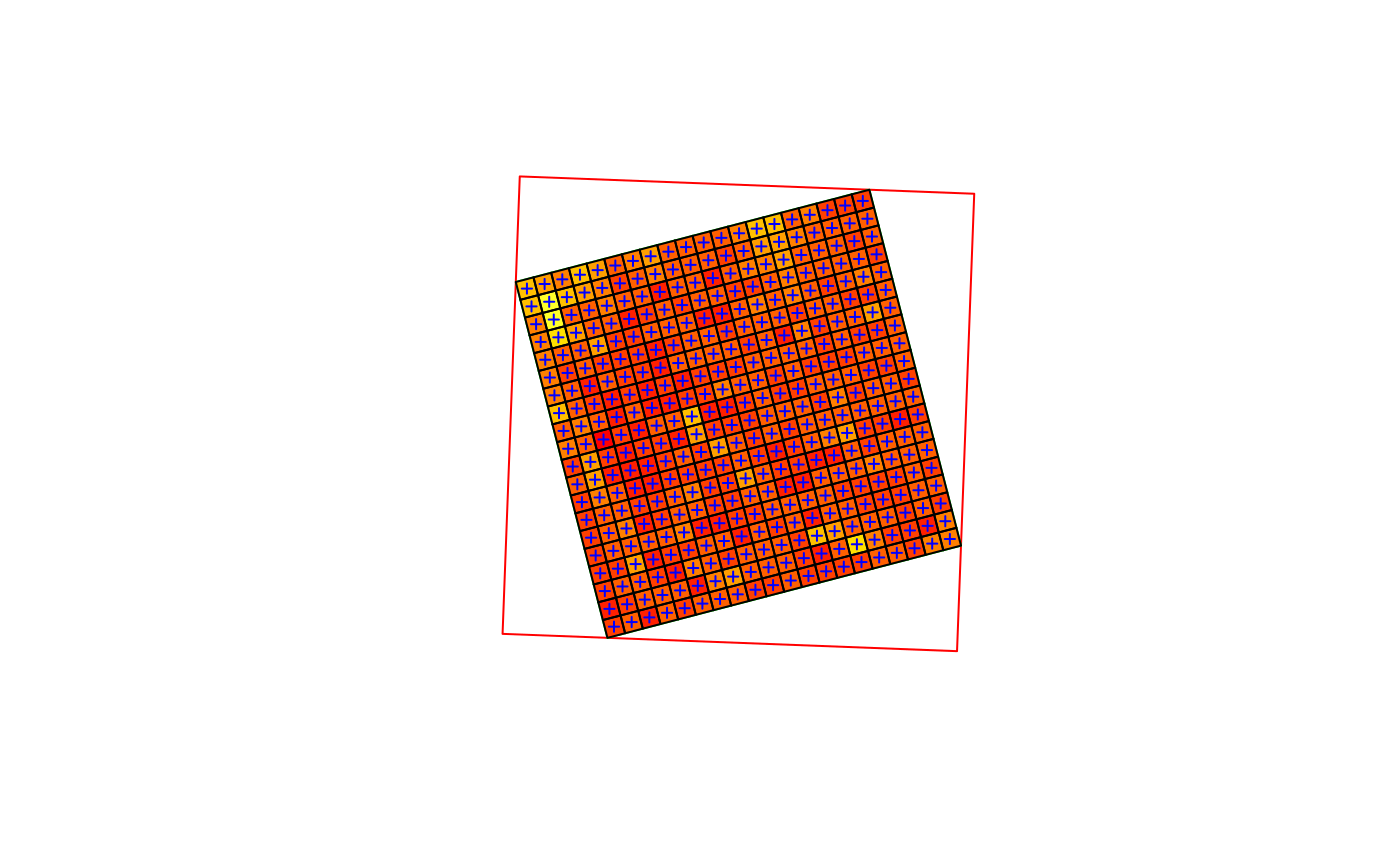transform geometries in stars objects to a new coordinate reference system, without warping

# S3 method for stars
st_transform(x, crs, ...)

# S3 method for stars
st_transform_proj(x, crs, ...)

## Arguments

x

object of class stars, with either raster or simple feature geometries

crs

object of class crs with target crs

...

ignored

## Details

For simple feature dimensions, st_transform is called, leading to lossless transformation. For gridded spatial data, a curvilinear grid with transformed grid cell (centers) is returned, which is also lossless. To convert this to a regular grid in the new CRS, use st_warp (which is in general lossy).

## Examples

geomatrix = system.file("tif/geomatrix.tif", package = "stars")
#> stars object with 2 dimensions and 1 attribute
#> attribute(s):
#>                Min. 1st Qu. Median    Mean 3rd Qu. Max.
#> geomatrix.tif    74     107    123 126.765     132  255
#> dimension(s):
#>   from to  offset delta                refsys point x/y
#> x    1 20 1841002   1.5 WGS 84 / UTM zone 11N  TRUE [x]
#> y    1 20 1144003  -1.5 WGS 84 / UTM zone 11N  TRUE [y]
#> sheared raster with parameters: -5 -5
new = st_crs(4326)
y = st_transform(x, new)
plot(st_transform(st_as_sfc(st_bbox(x)), new), col = NA, border = 'red')
plot(st_as_sfc(y, as_points=FALSE), col = NA, border = 'green', axes = TRUE, add = TRUE)
image(y, col = heat.colors(12), add = TRUE)
plot(st_as_sfc(y, as_points=TRUE), pch=3, cex=.5, col = 'blue', add = TRUE)
plot(st_transform(st_as_sfc(x, as_points=FALSE), new), add = TRUE)